# How Does Hashing Work in Blockchain?

In simple terms, hashing is a mathematical computation which can take any string as input and produce a completely different output of a fixed length. Let’s explore how hashing is used in blockchain in the article below!

Home » How Does Hashing Work in Blockchain?

In this article we will delve a bit deeper into what hashing is, why it’s important for blockchain and how it is used there. We will also demystify hashing by using a very simple example – a piece of data on which we will try to calculate a hash for illustration purposes. The outline of the article is as follows:

## How are blocks arranged in blockchain?

As discussed in the blockchain internals article, a blockchain is essentially a collection (or chain) of blocks.  A block in turn is just a structure that holds some data.

Admittedly this sounds rather oversimplified, so let’s explore what’s more to blocks via an example. Let’s assume we are dealing with financial data. In this case, a block would contain a collection of financial transactions, where each individual transaction would contain details such as which parties were involved in the transaction (the buyer and seller), the amount transacted, the date of the transaction and so on.

If we’d be dealing with a physical ledger, we would be writing all these details for each transaction into a ledger page. Whenever a page would be filled up, another page would be turned and started to be filled with the next transactions.

So a block can be thought as being just like a ledger page. Whenever a block is filled up, another block is added. And this next block is linked to the previous block. Blocks are added in this manner and form a sort of a “chain” of blocks, hence the name blockchain. So the blockchain is nothing more than a chain of blocks linked together.

Each block can store the details of hundreds to thousands of transactions. As new transactions occur, they are first added to what is called a transaction pool. Then during block creation, the  transactions get picked up from the pool and get batched together into that block. The block is then added after any existing blocks. Notice the chain concept here – the Blockchain grows by having more and more blocks appended to it, each of which is added in succession to the previous one.

New blocks can never be inserted in the middle. The blocks that are already in place can never be rearranged in some different order. And no previous block can be easily edited or deleted. Hence, the term “append only” is often used to describe Blockchain.

Building it as a chain makes it possible to keep both the current state of whatever data is being kept in the blockchain, as well as all the history that led to that point. However, the question arises, if this blockchain is actually shared across multiple computers (nodes), potentially even thousands of them, and they all have a copy of the same data, what would stop someone sitting behind one of those computers to take a block and change some transaction in it? Be it to add a new transaction, tamper with the details of an existing one, or delete transactions altogether? Glad that you asked. Let’s look at the answer to that.

## What is cryptographic hashing?

Every block in the Blockchain uses a cryptographic hash. Hashing isn’t something that is only specific to Blockchain. Cryptographic hashing, or simply hashing, is a typical programming activity that is especially present when dealing with security or encryption.

During hashing the original data is taken and broken down into small parts. Then, several calculations are done on those distinct parts, and then the parts are combined again in some way to form a result. A seemingly useless and random result that was actually based on the original data.

## A hashing example

Let’s go through a very simplified method of calculating a hash on a piece of data. The actual hashing algorithms are of course very different, but this will hopefully help clear the concept. Our data for this example will be the following sentence:

MrCryptoz makes blockchain easy

Now let us take every individual letter of this sentence and map it to a number based on the position of that letter in the English alphabet, so A would be 1, B would be 2 etc.

MrCryptoz makes blockchain easy translates to:

M – 13 th letter in the English alphabet

r – 18 th letter in the English alphabet

C – 3 rd letter in the English alphabet

…and so on.

Adding these numbers together, we have:

13+18+3+18+25+16+20+15+26  +  13+1+11+5+19  + 2+12+15+3+11+3+8+1+9+14 + 5+1+19+25 = 331

Now let’s take every second number and add them up. It gives us 169.

13+18+3+18+25+16+20+15+26  +  13+1+11+5+19  + 2+12+15+3+11+3+8+1+9+14 + 5+5+19+25 = 173

We’ll then take the remaining numbers and add all those up to give us 162.

13+18+3+18+25+16+20+15+26 13+1+11+5+19  + 2+12+15+3+11+3+8+1+9+14 + 5+1+19+25 = 162

Let’s take both of those results and just reverse them in place. We get 961 and 261

Now let’s multiply them. 961 x 261 = 250821.

Now we’ll convert every second number back to a letter. So 5 becomes an E, 8 becomes an H, and 1 becomes an A.

Our final result is 2E0H2A.

Does it look like we just made up several random and rather meaningless calculations?

We did indeed.

An actual hash is calculated in a more complicated way, but the principle to keep in mind is that the result that is obtained is completely dependent on the original piece of data. And that this result is consistent and repeatable, meaning that even 10 years from now, if we applied the same process to the same data, we’d get the exact same result.

And if we made even the smallest change to the original data, and applied the same process, same calculations, we’d get a completely different result. If we change one letter only of our example sentence, making the ‘a’ in blockchain an ‘e’, we would get an entirely different result:

MrCryptoz makes blockchein easy

translates to:

13+18+3+18+25+16+20+15+26  +  13+1+11+5+19  + 2+12+15+3+11+3+8+5+9+14 + 5+1+19+25 = 335

Now let’s take every second number and add them up. It gives us 173.

13+18+3+18+25+16+20+15+26  +  13+1+11+5+19  + 2+12+15+3+11+3+8+5+9+14 + 5+1+19+25 = 173

We’ll then take the remaining numbers and add all those up to give us 162.

13+18+3+18+25+16+20+15+26 13+1+11+5+19  + 2+12+15+3+11+3+8+1+9+14 + 5+1+19+25 = 162

Reversing the numbers gives us 371 and 261.

Their multiplication result is 371 x 261 = 96831.

After doing the final switching to letters of every second digit we get 9F8C1.

So although we only changed a single letter, the result changed completely, from 2E0H2A to 9F8C1.

Another property of hashing is that the result will be much much smaller than the original data. Instead of a sentence, we could hash a 100 page document and still end up with a short hash value.

It is also important to understand that hashing is not encryption. In encryption, the idea is to encrypt some data to get a scrambled and unreadable result. But that unreadable result can subsequently be decrypted to get the original data back. Hashing is different. It is not reversible. Having the hash value only, it is not possible to convert it back to the original data.

## Why is hashing used in blockchain?

Hashing in blockchain is used in order to act as a kind of fingerprint of the data. A way to verify that the original data hasn’t been changed or tampered with. So whenever you take the hash of the original data, it should match the hash that has been stored for that data. If the newly calculated hash does not match the previous one, it means that someone has tampered with the data and we are not dealing with the original anymore.

In blockchain, hashes are incorporated at several levels. First, each transaction in a block has its own hash based on that transaction’s contents only. Then, each block also has its unique hash for the block itself. Additionally, when a new block is formed, one of the pieces of data it keeps is a copy of the previous block’s hash value.

What this implies is that if you try to change any tiny piece of previous data in a blockchain, its associated hash will no longer be the same. So if one tries to change a transaction, the hash of that transaction will no longer be the same. And then also the hash of the block will no longer be the same, because the hash of the block was calculated on top of all transactions of that block. Further, even the next block, which had a copy of the hash, no longer matches. This causes a domino effect where none of the succeeding blocks match. So it is this cryptographic hashing that makes blockchain extremely resistant to alteration.Tampering with data in a block results in a changed hash for that block. The next block in the blockchain keeps a copy of the previous block’s hash, so it becomes evident by that mismatching hash that some data was tampered with

While we’re here, let’s quickly clarify something. You will often hear the term immutable used for Blockchain. Immutable as in unchangeable, meaning the data in a blockchain can’t be changed. But this is not entirely true. Technically, a block in the blockchain can be changed. It’s more that there’s really no point, because it will be obvious that the data has been tampered with as that block will no longer be consistent neither within itself or with all the other copies in the Blockchain network (due to the mismatching hashees). What’s important is that Blockchain is tamper-evident by design, which is the whole point of this technology.

And as we’ve seen already, multiple copies of the Blockchain ledger are distributed among many computers, so even if there is tampering with one of them, the other computers would have the intact copies. What happens in these cases is that Blockchain implements what’s called a consensus mechanism.

To simplify it, if 10 copies of the Blockchain ledger exist, seven out of which agree with each other based on those cryptographic hashes, there exists a consensus and that’s the version that will be then replicated across the other nodes in the network.

## Conclusion

This article provided a high-level, simplified overview of how cryptographic hashing is used on the blockchain. In layman’s terms, hashing is a method of taking some data and breaking it up into pieces, and then performing a set of calculations and transformations on those distinct pieces of data. Finally, the transformed pieces are combined back together to output a single result of a set width, according to the hashing algorithm used.

Cryptographic hashing is used on the blockchain in order to act as a sort of fingerprint of the data in a block. After a block is filled up with transactions, a hash is calculated for the entire block. If someone maliciously tries to tamper with even the slightest amount of the data in the block, the hash value would drastically change. This would make the tampering evident, so the hacker would not be able to get away with the change. Additionally, each consecutive block in a blockchain contains the hash value of the preceding block. So if the hash value of the previous block has been changed, this will become evident in the next block, making the data tampering evident.

If you are still intrigued by blockchain and its elegant characteristics that make it so worthy of all the excitement around it, make sure you check the other articles in our academy to delve a bit deeper into it. What we recommend is that you check out this article next, which outlines some of the most interesting and exciting use cases of blockchain.

What kinds of hashing algorithms are used on different types of blockchain?

SHA-256 is one of the most popular hashing algorithms used in different blockchain implementations. For example, Bitcoin uses  SHA-256 for its blockchain. The Ethereum blockchain currently uses the  Keccak-256 cryptographic hash function, but it has been announced that Ethereum 2.0 will also be using SHA-256.

Is hashing the same as encryption?

No, hashing is not the same as encryption. Encryption is used to make a piece of data scrambled and unreadable, but someone containing the encryption key, would be able to decrypt the encrypted data back to its original format.

Is hashing reversible?

No, hashing is not reversible. By only having the hash value, one cannot derive the original data that produced that hash value.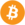Bitcoin
\$ 22,900.142.36%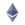Ethereum
\$ 1,621.373.39%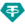Tether
\$ 1.000.01%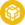BNB
\$ 326.021.56%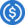USD Coin
\$ 1.000.05%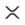XRP
\$ 0.3987643.85%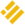Binance USD
\$ 0.9997910.04%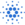Cardano
\$ 0.3872534.02%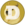Dogecoin
\$ 0.0917695.68%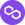Polygon
\$ 1.206.27%